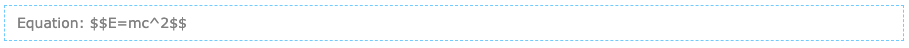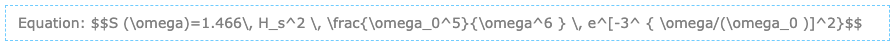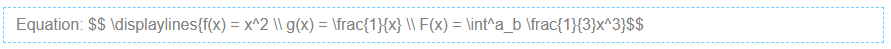# Equations with LaTeX

Mathematical equations may be created using LaTeX notation [https://edtechbooks.org/-qYV].

While editing a chapter, select Tools > LaTeX Equation and type in the formula. Upon submitting, you will see a box in the editor that looks like the following:You can continue to edit the formula in the editor (provided that you do not remove the double-\$ symbols). When saved, the example equation will render as this:

$$E=mc^2$$

Here is a more sophisticated example:Which will render like this:

$$S (\omega)=1.466\, H_s^2 \, \frac{\omega_0^5}{\omega^6 } \, e^[-3^ { \omega/(\omega_0 )]^2}$$

For multi-line equations, use a double-slash (\\) to separate each line as follows:$$f(x) = x^2 \\ g(x) = \frac{1}{x} \\ F(x) = \int^a_b \frac{1}{3}x^3$$

If you need assistance writing LaTeX equations, there are many online tools that can help you do this such as the Online LaTeX Equation Editor [https://edtechbooks.org/-NgK].

All rendering is performed courtesy of MathJax [https://www.mathjax.org/] and CodeDogs [https://edtechbooks.org/-IDD].